TYPE 70: PHOTOVOLTAIC-cell/module/array

General Description

TYPE 70 models a single solar cell or a PV module or an array consisting of a number of modules. It uses the one-diode model. The cell/module is described by cell parameters. No partial shading effects are taken into account by this component. The user may specify, if a voltage is forced upon the cell/module (e.g. by a battery) or if maximum power point tracking (MPPT) is performed. The MPPT is part of the TYPE 70 component. For the treatment of the cell temperature 4 modes can be chosen (see documentation for details):

tcmod mode for calculation of cell temperature
0: cell temperature tc is input
1: tc calculated with simple energy balance
2: tc calculated with more accurate energy balance according to /1/,/2/
3: like 2, but with phase change material (PCM)attached to the back side of the cell

The sky temperature in tcmod 2 and 3 is calculated using the model of /3/ for the clear sky and a constant temperature difference between ambient and sky for an overcast sky.

The first input to the component is a switch (a control signal). This is useful for PV systems that may require to turn the array off, e.g. in order to protect a battery from being overcharged.

Nomenclature

ab - solar radiation absorption of module
"b - combined heat transfer coefficient back side
"f - combined heat transfer coefficient front side
Am - module area (total)
Az - cell area (per cell or total per module)
\$ - array tilt angle
boltz - Boltzmann constant
Cdist - factor for distance cell - roof (0 = no distance, 1 = huge distance)
cl - specific heat capacity of liquid phase change material (PCM)
cs - specific heat capacity of solid PCM
c1 - ik0*temp.coefficient
c2 - Standard Temperature
deltt - time step
diod - diode quality factor
dPCM - thickness of PCM (solid)
echg - electron charge
egap - Band Gap Energy
, - glass emisivity
eps - relative tolerance for Newton's and secant method
err - relative error in iteration
eta - efficiency
fc - function for secant method iteration
( - factor for sky overcast (0 = no overcast, 1 = total overcast)
gtot - radiation transformed into heat
hPCM - latent heat of melting (PCM)
hf - convectiv heat transfer coefficient (front), based on /2/
hb - convectiv heat transfer coefficient (backside)
Icell - current through photovoltaic cell
Ik - approximation of short circuit current
Ik0 - Standard Short Circuit Current/Standard Irradiation
info - TRNSYS info array
Is - dark current
Isc - short circuit current
Is0 - Dark Current Factor [A/K3]
IT - Total incident radiation on a flat surface per unit area
kapalt - old portion of liquid PCM (0 = all solid, 1 = all liquid)
mode - >0:array,<0:no array, |mode|=1:cell-, =2:module-par.
mp - number of module series in parallel in the array
mpp - MPPT flag
ms - number of modules in series in the array
np - number of cell series in parallel (per module)
ns - number of cells in series (per module)
out - TRNSYS output vector
par - TRNSYS parameter vector
Pel - electric power
pelo - power of last iteration step
f min - minimal incident radiation for which calculation is made [W/m2]
qmalt - heat used for melting exclusive this time step
DPCM - density of PCM (solid)
rpar - parallel resistance for model
rser - series resistance of model
F - Stefan-Boltzmann constant
Tamb - ambient temperature
tau - relative tolerance for successive substitution
Tcell - cell temperature
Told - old cell temperature
Tmelt - melting temperature of PCM
Troom - room temperature (backside of cell)
Tsky - sky temperature
Ucell - voltage
Ucc - voltage for one cell saved for iterations
Uoc - open circuit voltage
Uroof - u-value (roof)
U0g - first guess for open circuit voltage
Vwind - wind speed
xin - TRNSYS input vector

Mathematical Description

Approximation of short circuit current:Dark current: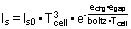I-U equation: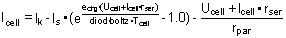For the temperature calculation of the photovoltaic cells there are three modes. It is also possible to give the temperature as input (for example if measurements have been done and the model is verified) in mode 0.

mode 1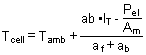mode 2

The total radiation on the solar cell that is turned into heat: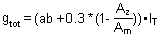The sky temperature is calculated: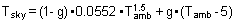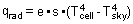Heat loss from the front side of the photovoltaic cell: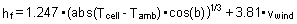Heat loss from the back side of the photovoltaic cell: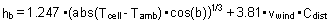or if the modules are very close to the surface under (the roof):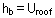The cell temperature is calculated from the heat balance: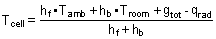mode 3

The heat losses and gains are calculated as in mode 2. In addition the melting of the phase change material (PCM) is taken into account. The calculations are based on the heating capacities for the PCM as solid and as liquid. The calculations will also keep track of how much of the PCM that are melted. This is based on the melting energy for the PCM.

TRNSYS Component Description

PARAMETER NO. DESCRIPTION

1 mode - +1: array (modepar=2), -1: no array (modepar=0)
2 aream - module area (total)
3 areaz - cell area (per cell or total per module)
4 ns - number of cells in series (per module)
5 np - number of cell series in parallel (per module)
mode = 1
6 ms - number of modules in series in the array
7 mp - number of module series parallel in the array
all modes
6 + modpar tcmod - mode for calculation of cell temperature;
0: cell temperature tc is input
1: tc calculated with simple energy-balance
2: tc calculated with accurate energy-balance
3: equal 2, but with phase change material
tcmod = 1
7 + modpar alf - combined heat transfer coefficient front side
8 + modpar alb - combined heat transfer coefficient back side
tcmod = 2,3
7 + modpar abfak - factor for distance cell - roof (0 = no distance, 1 = huge distance). abfak<10-8 => abfpar=1, else abfpar=0
8 + modpar kwert - U-value (roof)
8 + modpar + abfpar epsgla - glass emisivity
9 + modpar + abfpar Tstart - tc at beginning of simulation
tcmod=3
10 + modpar + abfpar Tmelt - melting temperature of PCM
11 + modpar + abfpar cs - specific heat capacity of solid PCM
12 + modpar + abfpar cl - specific heat capacity of liquid PCM
13 + modpar + abfpar dPCM - thickness of PCM (solid)
14 + modpar + abfpar hPCM - latent heat of melting (PCM)
15 + modpar + abfpar kPCM - density of PCM (solid)

INPUT NO. DESCRIPTION

• 1 ypv - control function (switch)
• 2 IT - incident radiation
• 3 Uc - voltage for one cell
• 4 Tamb - ambient temperature
• 5 \$ - array tilt angle for tcmod = 0:
• 6 Tc - cell temperature for tcmod = 2,3:
• 6 ( - factor for sky overcast (0 = clear sky, 1 = totally overcast)
• 7 Vwind - wind speed
• 8 Troom - room temperature (backside of cell)

OUTPUT NO. DESCRIPTION

• 1 Uc - voltage over one cell
• 2 Ic - current from one cell times the fraction of cells connected to load
• 3 Pel - electric power from one cell times the fraction of cells connected to load
• 4 Tc - temperature of cell in degrees Celsius
• 5 Z - efficiency of panel
• 6 Uoc - Open circuit voltage of one cell
• 7 Isc - Short circuit current through one cell
• 8 ypv - Fraction of cells in panel connected to load
•

Informational Flow Diagrams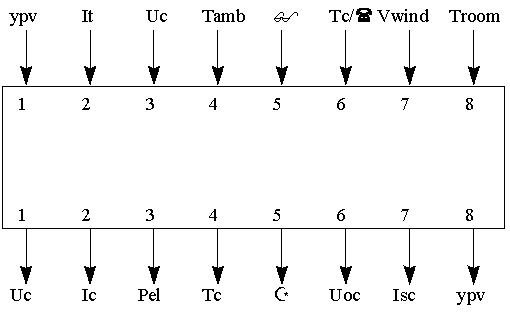Data file input

The data for a Photovoltaic cell have to be given in a separate file. The first line in this file will give the number of photovoltaic cells given in the file. Each different cell will have two lines; the first line contains the number of the cell in the file and the name of the cell, the second line contains the data for the cell. The data file for the photovoltaic cell will be like this (the third line (P1-P9) should not be in the file, but are included here to illustrate what each data represents):

1,Solarcell
0.0078 142.59 0.002996 279986 0 0 1.12 1.47 0.80
P1 P2 P3 P4 P5 P6 P7 P8 P9

P1 = Rser : series resistance of model
P2 = Rpar : parallel resistance for model
P3 = Ik0 : Standard Short Circuit Current/Standard Irradiation
P4 = Is0 : Dark Current Factor [A/K3]
P5 = C1 : ik0*temp.coefficient
P6 = C2 : Standard Temperature
P7 = Egap : Band Gap Energy
P8 = Diod : diode quality factor
P9 = ab : solar radiation absorption of module

OUTPUT to file logical unit 6 will look like this:

Parameters from file to PVcell, unit xx:
rser = 7.8000E-03 rpar = 1.4259E+02 ik0 = 2.9960E-03
is0 = 2.7999E+05 c1 = 0.0000E+00 c2 = 2.7315E+02
egap = 1.1200E+00 diod = 1.4700E+00 ab = 8.0000E-01

References

/1/ Sand '79-7056', report Sandia Lab
/2/ J.W. Stultz, L.C. Wen, JPL Document No. 5105-31, 1977
/3/ Duffie, J.A., Beckmann, W.A., Solar Engineering of Thermal Processes, John Wiley & Sons, 1980